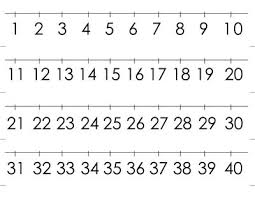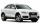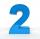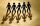# The ratio

The ratio of two numbers is 4, and their sum is 75. Determine both numbers.

a =  60
b =  15

### Step-by-step explanation:

a=4b
a+b=75

a=4•b
a+b=75

a-4b = 0
a+b = 75

a = 60
b = 15

Our linear equations calculator calculates it.Did you find an error or inaccuracy? Feel free to write us. Thank you!Tips to related online calculators
Do you have a linear equation or system of equations and looking for its solution? Or do you have a quadratic equation?

## Related math problems and questions:

• Two numbersThe difference between the two numbers is 74. If we divide a larger number by a smaller one, we get a quotient 7 and the rest of 2. Determine both numbers.
• Ratio of two unknown numbersTwo numbers are given. Their sum is 30. We calculate one-sixth of a larger number and add to both numbers. So we get new numbers whose ratio is 5:7. Which two numbers were given?
• Five numbers in ratioFive integers are in the ratio 1: 2: 3: 4: 5. Their arithmetic mean is 12. Determine the smallest of these numbers.
• The sum 15The sum of the real numbers x and y is 24. Their difference is 12. What is the value of xy?
• The sum 9The sum of two integers is 4. Their difference is 8. What are this two integers?
• Ratio three numbersThree numbers SUV are in the ratio 1:2:3. Their sum is 24. Find this numbers and write their add and sum.
• Sum and ratioThe sum and ratio of two numbers is equal to 10. Which numbers are they?
• The sumThe sum of five consecutive odd numbers is 75. Find out the sum of the second and fourth of them.
• In aIn a triangle, the aspect ratio a: c is 3: 2, and a: b is 5: 4. The perimeter of the triangle is 74cm. Calculate the lengths of the individual sides.
• Three numbersFind three numbers so that the second number is 4 times greater than the first and the third is lower by 5 than the second number. Their sum is 67.
• Numbers at ratioThe two numbers are in a ratio 3:2. If we each increase by 5 would be at a ratio of 4:3. What is the sum of original numbers?
• FriendsSome friends had to collect the sum 72 EUR equally. If the three refused their part, others would have to give each 4 euros more. How many are friends?
• The sumThe sum of the squares of two immediately following natural numbers is 1201. Find these numbers.
• The largerThe larger of two numbers is nine more than four times the smaller number. The sum of the two numbers is fifty-nine. Find the two numbers.
• AS sequenceIn an arithmetic sequence is given the difference d = -3 and a71 = 455. a) Determine the value of a62 b) Determine the sum of 71 members.
• Sum of the seventeen numbersThe sum of the 17 different natural numbers is 154. Determine the sum of the two largest ones.
• Two numbers 7The sum of two consecutive even numbers is 30. Find the numbers.